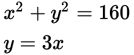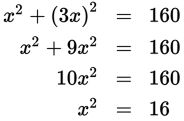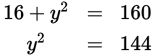# SAT Math Multiple Choice Question 929: Answer and Explanation

### Test Information

Question: 92913. If (x, y) is a solution to the system of equations above, what is the value of y2?

• A. 12
• B. 16
• C. 120
• D. 144

Explanation:

D

Difficulty: Medium